(原創) 如何處理signed integer的加法運算與overflow? (SOC) (Verilog)

Abstract

Introduction

Verilog在宣告reg與wire時，雖然能使用+ – * /，並合成出相對的加法器、乘法器與除法器，但這些都是無號數(unsigned integer)運算，也就是說只能做大於或等於0的整數加減乘除運算，無法處理負數運算；除此之外，不像C/C++的int就是32 bit，為了節省硬體cost，我們會根據值域，小心的宣告reg與wire的bit數，如只有4 bit或8 bit而已，這樣經過運算後，可能在某個boundary test pattern下，一不小心就overflow了。

(原創) 無號數及有號數的乘加運算電路設計 (IC Design) (Verilog) (OS) (Linux)(原創) 如何設計乘加電路? (SOC) (Verilog) (MegaCore)中，我都曾經討論過這個問題，這次打算更仔細重新討論，並將overflow議題一並考慮。

Verilog的運算
Verilog所提供的運算分unsigned與signed兩種：

• Unsigned：不含signed bit
• 以4 bit來說，值域從0000~1111，也就是0 ~ 15
• Signed：含signed bit(MSB為signed bit，1為負，0為正，負數使用2補數表示)
• 以4 bit來說，值域從1000~0111，也就是-8 ~ +7

Normal Condition (沒有Overflow)
(+6) + (-3) = (+3)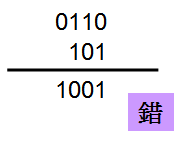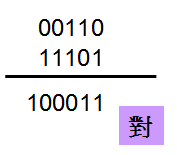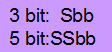Boundary Condition (正Overflow)
(+7) + (+3) = (+10)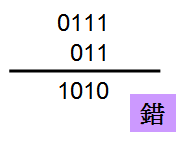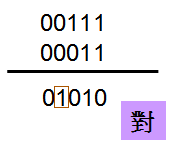Boundary Condition (負Overflow)
(-5) + (-4) = (-9)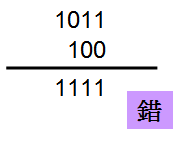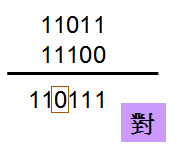• m bit + m bit => (m+1) bit
• m bit + n bit => (m+1) bit，其中n < m
• m bit與n bit都必須先做signed extension到(m+1) bit才能相加
• 若結果有到(m+2) bit則忽略之，實際的結果為(m+1) bit
• 若Sum[m+1] ^ Sum[m]為1，表示有overflow
• 若Sum[m+1]為0且Sum[m]為1，則為正overflow
• 若Sum[m+1]為1且Sum[m]為0，則為負overflow1 /*
2 (C) OOMusou 2009 http://oomusou.cnblogs.com
3
4 Filename    : signed_add.v
5 Simulator   : NC-Verilog 5.4 + Debussy 5.4 v9
6 Description : signed add & overflow
7 Release     : Oct/24/2009 1.0
8 */
9
10 module signed_add (
11   clk,
12   rst_n,
13   a_i,
14   b_i,
15   sum_o
16 );
17
18 input clk;
19 input rst_n;
20 input [3:0] a_i;
21 input [2:0] b_i;
22 output [3:0] sum_o;
23
24 reg [4:0] sum_t;
25 always@(posedge clk or negedge rst_n)
26   if (~rst_n)
27     sum_t <= 5'h0;
28   else
29     sum_t <= {a_i, a_i} + {{2{b_i}}, b_i};
30
31 assign sum_o = (~sum_t &  sum_t) ? 4'b0111 : // + overflow
32                ( sum_t & ~sum_t) ? 4'b1000 : // - overflow
33                sum_t[3:0];
34 endmodule

20 ~ 22行input [3:0] a_i;
input [2:0] b_i;
output [3:0] sum_o;

29行sum_t <= {a_i, a_i} + {{2{b_i}}, b_i};

31行(~sum_t &  sum_t) ? 4'b0111 : // + overflow

32行( sum_t & ~sum_t) ? 4'b1000 : // - overflow

Testbench1 /*
2 (C) OOMusou 2009 http://oomusou.cnblogs.com
3
4 Filename    : signed_add_tb.v
5 Simulator   : NC-Verilog 5.4 + Debussy 5.4 v9
6 Description : signed add & overflow testbench
7 Release     : Oct/24/2009 1.0
8 */
9
11
13
14 reg clk;
15 reg rst_n;
16 reg [3:0] a_i;
17 reg [2:0] b_i;
18 wire [3:0] sum_o;
19
20 // 4 bit
21 // -8 ~ +7
22 // 3 bit
23 // -4 ~ +3
24 initial begin
25   //a_i <= 4'b0000;
26   //b_i <= 3'b000;
27   a_i <= 4'd0;
28   b_i <= 3'd0;
29
30   // normal
31   // (+6) + (-3)
32   #10;
33   //a_i <= 4'b0110;
34   //b_i <= 3'b101;
35   a_i <= 4'd6;
36   b_i <= -3'd3;
37
38   // overflow
39   // 7 + 3 = 10
40   #20;
41   //a_i <= 4'b0111;
42   //b_i <= 3'b011;
43   a_i <= 4'd7;
44   b_i <= 3'd3;
45
46   // underflow
47   // (-5) + (-4)
48   #20;
49   //a_i <= 4'b1011;
50   //b_i <= 3'b100;
51   a_i <= -4'd5;
52   b_i <= -3'd4;
53
54   #20;
55   //a_i <= 4'b0000;
56   //b_i <= 3'b000;
57   a_i <= 4'd0;
58   b_i <= 3'd0;
59 end
60
61
62 initial clk = 1'b0;
63 always #10 clk = ~clk;
64
65 initial begin
66   rst_n = 1'b0;
67   #5;
68   rst_n = 1'b1;
69 end
70
71 initial begin
72   $fsdbDumpfile("signed_add.fsdb"); 73$fsdbDumpvars(0, signed_add_tb);
74   #100;
75   \$finish;
76 end
77
79   .clk(clk),
80   .rst_n(rst_n),
81   .a_i(a_i),
82   .b_i(b_i),
83   .sum_o(sum_o)
84 );
85
86
87 endmodule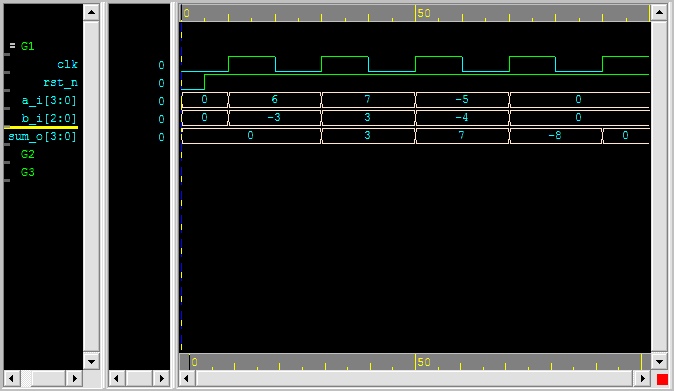7 + 3 = 10，因為已經正overflow，所以使用4 bit最大值+7表示‧
(-5) + (-4) = (-9)，因為已經負overflow，所以使用4 bit最小值-8表示‧

Conclusion

posted on 2009-10-31 10:48 真 OO无双 阅读(...) 评论(...) 编辑 收藏

• 随笔 - 914
• 文章 - 0
• 评论 - 4357
• 引用 - 161